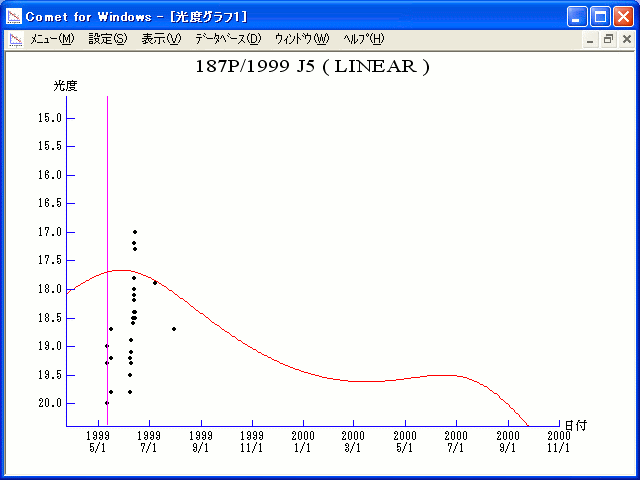# \$B%j%K%"WB@1(B

187P/LINEAR (1999)###\$B%W%m%U%#!<%k(B

 \$BId9f(B 187P/1999 J5 \$BH/8+F|(B 1999\$BG/(B 5\$B7n(B12\$BF|(B \$BH/8+8wEY(B 19.0\$BEy(B \$BH/8+ Lincoln Laboratory Near-Earth Asteroid Research project

###\$B50F;MWAG(B

```                    Epoch = 1999 May  22.0 TT
T = 1999 May  12.1310 TT         Peri. = 132.1799
e = 0.169470                     Node  = 112.0187  2000.0
q = 3.712711 AU                  Incl. =  13.7164
a =  4.470289 AU    n = 0.1042799    P =   9.45 years
```

###\$B@1?^(B###\$B8wEYJQ2=(B

```        m1 = -13.0 + 5 log\$B&\$(B + 50.0 log r
```##### \$B50F;MWAG\$O(B G. V. Williams \$B\$N7W;;\$K\$h\$k\$b\$N\$G!"(B IAUC 8827 \$B\$K7G:\\$5\$l\$?\$b\$N\$G\$9!#(B \$B@1?^\$O(B StellaNavigator Ver.2.0 for Windows (\$B%"%9%H%m%"!<%D(B \$BJTCx(B / \$B%"%9%-!<=PHG6I4)(B) \$B\$G:n@.\$7\$?\$b\$N\$G\$9!#(B \$B8wEY%0%i%U\$O(BComet for Windows\$B\$G:n@.\$7\$?\$b\$N\$G\$9!#(B